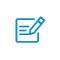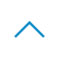•• 注册 忘记密码?

# Attention！当推荐系统遇见注意力机制

DIN和DIEN都是阿里针对CTR预估的模型，都主要是对用户历史行为数据的进一步挖掘的工作。CTR预估任务是，根据给定广告/物品、用户和大量的上下文情况等信息，对点击进行预测，所以对用户的兴趣理解，历史行为数据非常重要。

DIN

DIN这篇文章的Attention故事出发点在于两点观察：
「Diversity」：多样性是指用户的兴趣是广泛的，一个用户会对多个物品，多个领域感兴趣
「Local activation」：部分对应是指只有部分历史数据与目前推荐的物品相关（如推荐零食物品就与用户以前买过什么装备无关）

「1. Data Dependent Activation Function（Dice激活函数）」

def dice(_x, axis=-1, epsilon=0.000000001, name=''):
#Data Adaptive Activation Function
with tf.variable_scope(name_or_scope='', reuse=tf.AUTO_REUSE):
alphas = tf.get_variable('alpha'+name, _x.get_shape()[-1],
initializer=tf.constant_initializer(0.0),
dtype=tf.float32)
beta = tf.get_variable('beta'+name, _x.get_shape()[-1],
initializer=tf.constant_initializer(0.0),
dtype=tf.float32)
input_shape = list(_x.get_shape())

reduction_axes = list(range(len(input_shape)))
del reduction_axes[axis]
broadcast_shape =  * len(input_shape)
broadcast_shape[axis] = input_shape[axis]

# case: train mode (uses stats of the current batch)
#计算batch的均值和方差
mean = tf.reduce_mean(_x, axis=reduction_axes)
brodcast_mean = tf.reshape(mean, broadcast_shape)
std = tf.reduce_mean(tf.square(_x - brodcast_mean) + epsilon, axis=reduction_axes)
std = tf.sqrt(std)
brodcast_std = tf.reshape(std, broadcast_shape)
x_normed = tf.layers.batch_normalization(_x, center=False, scale=False, name=name, reuse=tf.AUTO_REUSE)
# x_normed = (_x - brodcast_mean) / (brodcast_std + epsilon)
x_p = tf.sigmoid(beta * x_normed)

return alphas * (1.0 - x_p) * _x + x_p * _x #根据原文中给的公式计算

def parametric_relu(_x):
#PRELU激活函数，形式上和leakReLU很像，只是它的alpha可学习
#alpha=0，退化成ReLU。alpha不更新，退化成Leak
with tf.variable_scope(name_or_scope='', reuse=tf.AUTO_REUSE):
alphas = tf.get_variable('alpha', _x.get_shape()[-1],
initializer=tf.constant_initializer(0.0),
dtype=tf.float32)
pos = tf.nn.relu(_x)
neg = alphas * (_x - abs(_x)) * 0.5 #用alpha控制

return pos + neg

「2. Adaptive Regularization（自适应正则）」

DIN的设计对工业界的帮助更大，因为上线的时候受制于内存所以User Embedding不能很大，那么显然无法很好的表示用户特征，想表示用户的多兴趣（多峰）就很难了。此时DIN基于用户历史行为再加入Attention就很好的缓解了这个问题。

DINE

Interest Extractor Layer

def auxiliary_loss(self, h_states, click_seq, noclick_seq, mask, stag = None):
mask = tf.cast(mask, tf.float32)
click_input_ = tf.concat([h_states, click_seq], -1) #正例
noclick_input_ = tf.concat([h_states, noclick_seq], -1) #负例
#输到网络得到概率
click_prop_ = self.auxiliary_net(click_input_, stag = stag)[:, :, 0]
noclick_prop_ = self.auxiliary_net(noclick_input_, stag = stag)[:, :, 0]
#计算loss
click_loss_ = - tf.reshape(tf.log(click_prop_), [-1, tf.shape(click_seq)]) * mask
noclick_loss_ = - tf.reshape(tf.log(1.0 - noclick_prop_), [-1, tf.shape(noclick_seq)]) * mask
loss_ = tf.reduce_mean(click_loss_ + noclick_loss_)
return loss_
#辅助网络的结构
def auxiliary_net(self, in_, stag='auxiliary_net'):
bn1 = tf.layers.batch_normalization(inputs=in_, name='bn1' + stag, reuse=tf.AUTO_REUSE)
dnn1 = tf.layers.dense(bn1, 100, activation=None, name='f1' + stag, reuse=tf.AUTO_REUSE)
dnn1 = tf.nn.sigmoid(dnn1)
dnn2 = tf.layers.dense(dnn1, 50, activation=None, name='f2' + stag, reuse=tf.AUTO_REUSE)
dnn2 = tf.nn.sigmoid(dnn2)
dnn3 = tf.layers.dense(dnn2, 2, activation=None, name='f3' + stag, reuse=tf.AUTO_REUSE)
y_hat = tf.nn.softmax(dnn3) + 0.00000001
return y_hat

Interest Evolution Layer

interest drift：用户的兴趣具有偏向性。
interest individual：用户的兴趣之间具有独立性。

BST

item里面的蓝色是位置编码，红色是行为序列中的物品

BERT4Rec

PRM

Transformer的self-attention可以有效捕捉特征间的交互Copyright©2005-2020 Sykv.com 可思数据 版权所有    京ICP备14056871号-1扫码入群返回顶部